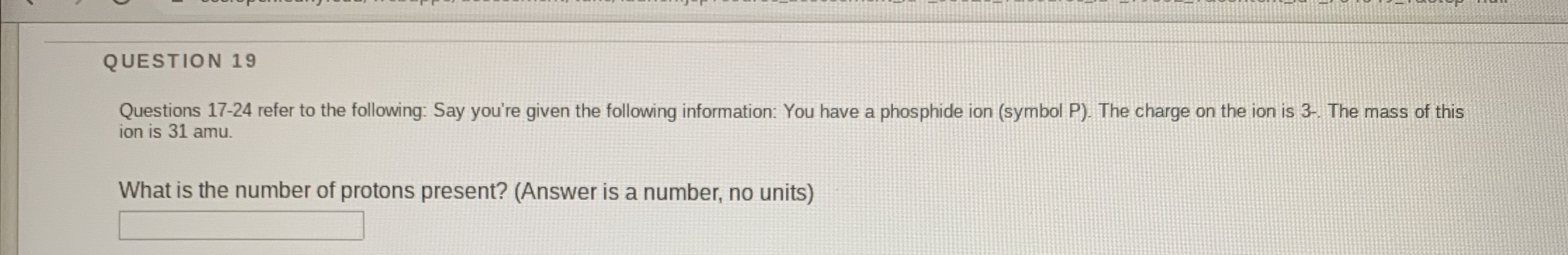# QUESTION 19Questions 17-24 refer to the following: Say you're given the following information: You have a phosphide ion (symbol P). The charge on the ion is 3- The mass of thision is 31 amu.What is the number of protons present? (Answer is a number, no units)

Question
4 viewshelp_outlineImage TranscriptioncloseQUESTION 19 Questions 17-24 refer to the following: Say you're given the following information: You have a phosphide ion (symbol P). The charge on the ion is 3- The mass of this ion is 31 amu. What is the number of protons present? (Answer is a number, no units) fullscreen
check_circle

Step 1

The given ion is phosphide ion and its charge is 3- that is P3- = P + 3e-

The number of electrons present in P is 15. Hence, the number of electrons present in pho...

### Want to see the full answer?

See Solution

#### Want to see this answer and more?

Solutions are written by subject experts who are available 24/7. Questions are typically answered within 1 hour.*

See Solution
*Response times may vary by subject and question.
Tagged in